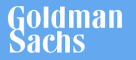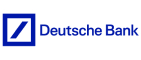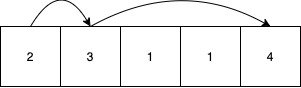New update is available. Click here to update.
Topics

# Jump Game

Moderate0/80
Average time to solve is 15m+22 more companies

## Problem statement

You have been given an array 'ARR' of ‘N’ integers. You have to return the minimum number of jumps needed to reach the last index of the array i.e ‘N - 1’.

From index ‘i’, we can jump to an index ‘i + k’ such that 1<= ‘k’ <= ARR[i] .

'ARR[i]' represents the maximum distance you can jump from the current index.

If it is not possible to reach the last index, return -1.

Note:
``````Consider 0-based indexing.
``````
Example:
``````Consider the array 1, 2, 3, 4, 5, 6
We can Jump from index 0 to index 1
Then we jump from index 1 to index 2
Then finally make a jump of 3 to reach index N-1

There is also another path where
We can Jump from index 0 to index 1
Then we jump from index 1 to index 3
Then finally make a jump of 2 to reach index N-1

So multiple paths may exist but we need to return the minimum number of jumps in a path to end which here is 3.
``````
Detailed explanation ( Input/output format, Notes, Images )
Sample Input 1:
``````5
2 3 1 1 4
``````
Sample Output 1:
``````2
``````
Explanation of sample input 1 :``````Consider the above figure:
We are initially at index 0, ARR is 2 which represents we can jump a maximum of 2 steps.

We jump 1 index from 0 to 1. At index 1, 'ARR' is 3 which represents we can jump a maximum of 3 steps so we jump 3 indices from 1 to 4 to reach the last index. Hence we return 2.

It can be proved that the end can't be reached in less than 2.
``````
Sample Input 2:
``````5
3 2 1 0 1
``````
Sample Output 2:
``````-1
``````
Constraints:
``````1 <= N <= 10 ^ 4
1 <= ARR[i] <= 10 ^ 4

Where ‘ARR[i]’ denotes the ‘i-th’ element of the ‘ARR’.

Time limit: 1 sec.
``````Console